# ChIPS - creating and using multiple plotsChIPS Threads (CIAO 4.11 ChIPS v1)

## Overview

#### Synopsis:

This thread demonstrates some of the capabilities for creating multiple plots in ChIPS; this includes creating a set of plots for use as a "strip chart" where the plots share a common axis, adjusting the spacing and relative sizes of plots, adding new plots to an existing figure, switching the order of plots within a grid, and customizing the visibility and position of labels.

Last Update: 15 Dec 2015 - Updated for CIAO 4.8.

## Introduction

The Starting ChIPS thread describes how to start ChIPS. Please see the ChIPS GUI section of that thread for information on the ChIPS GUI.

The data used in this thread is available in the chips_data.tar.gz file.

```unix% ls -1 chips/grids/
bax.fits
```

The plots used in this thread are based on the data in the FITS file bax.fits which contains the contents of the BAX Galaxy Cluster Database. This was generated from the ASCII file used in the symbol support for curves thread by

```unix% dmcopy "bax.lis[opt sep=|][BAX]" bax.fits
```

## Creating a strip chart

The strip_chart command is used to create a single column or row of plots that share a common axis. In this example we are going to use it to plot up flux, luminosity, and temperature values against redshift.

First we set up some preferences since we know what style of curve we want (we assume that the plots are being created in a new ChIPS session and so preferences that may have been used in other threads have not been changed).

```chips> pr = get_preferences()
chips> pr.curve.line.style = "noline"
chips> pr.curve.symbol.style = "square"
chips> pr.curve.symbol.size = 2
chips> set_preferences(pr)
```

We now use the strip_chart command to create three plots arranged vertically. We also show how the info.current preference setting can be used to indicate the current set of objects in the output of the info command (in the output below bold text is used to indicate the reverse video shown on screen):

```chips> strip_chart(3)
chips> print(get_preference("info"))
info.coord             : false
info.current           : false
info.depth             : false

chips> set_preference("info.current", "true")
chips> print(info())
Window [win1]
Frame [frm1]
Plot [plot1]   (0.15,0.65)  .. (0.90,0.90)
Border bottom [bx1]  top [bx2]  left [by1]  right [by2]
X Axis [ax1]
Y Axis [ay1]
Plot [plot2]   (0.15,0.40)  .. (0.90,0.65)
Border bottom [bx1]  top [bx2]  left [by1]  right [by2]
X Axis [ax1]
Y Axis [ay1]
Plot [plot3]   (0.15,0.15)  .. (0.90,0.40)
Border bottom [bx1]  top [bx2]  left [by1]  right [by2]
X Axis [ax1]
Y Axis [ay1]

chips> set_axis("all", ["color", "red"])
```

The axes of the top plot (as shown in Figure 1) are drawn in red to indicate that this is the current plot after the call to strip_chart. This means that any curve created by add_curve will be added to this plot (until the current plot is changed).

### Figure 1: A strip chart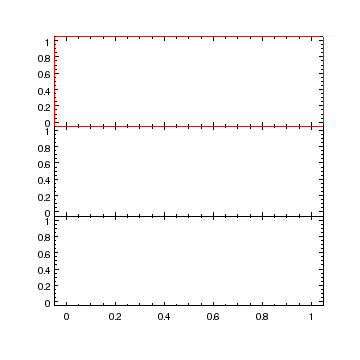### Figure 1: A strip chart

The strip_chart call has created three plots, arranged vertically, with no gaps between them. The axes have been set to the default range of 0 to 1 (adjusted by the value of the axis.pad preference setting of 0.05). The first (top) plot - called plot1 - is current, and is indicated by the red axes (from the call to set_axis).

Note that there are no X-axis ticklabels - i.e. the numeric values printed along an axis - for the top two plots. These were automatically hidden by the strip_chart call.

The output of the info command includes the position of the plots within the frame: the coordinates refer to the bottom-left and top-right corners, with (0,0) being the bottom-left corner and (1,1) the top-right corner of the frame.

We now fill in the first plot, after first removing the red axes using the undo command, set the scaling of the axes, and add a plot title and label to the Y axis. Since the X axis scale has been changed to logarithmic, the X axes in the other two plots has also changed to match. This is because the strip_chart command automatically "binds together" the shared axis so that changes in one plot - such as scaling or axis limits - will automatically be reflected in all plots (but only for the shared axis), as shown in Figure 2

```chips> undo()
chips> add_curve("bax.fits[cols z,fx]")
chips> log_scale()
chips> set_plot_ylabel("f_x (10^{-11} erg cm^{-2} s^{-1})")
chips> set_plot_title("BAX database")
```

### Figure 2: Filling in the first plot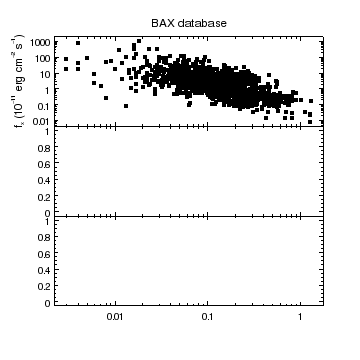### Figure 2: Filling in the first plot

Since the top plot was current the redshift-flux curve was added there. The X axis of all three plots has been changed to match the data (i.e. covers a range of roughly 0.002 to 1.5) since these axes have been bound together by the strip_chart call. The Y axes of the three plots are not bound together and so have independent ranges and scales.

The Y axis label shows off some of the LaTeX support available in ChIPS (in this case super- and sub-scripts).

To add a curve to a different plot - in this case the redshift-luminosity data in the middle plot - we need to change the current plot. The output of the info command earlier showed that the middle plot was called plot2, so we can use the current_plot command with this name. We chose to just change the scaling of the Y axis here, since the X axis is already drawn with a log scale (in this particular case we could have just called log_scale with no arguments and got the same result). The output of the following commands is shown in Figure 3.

```chips> current_plot("plot2")
chips> add_curve("bax.fits[cols z,lx]")
chips> log_scale(Y_AXIS)
chips> set_plot_ylabel("L_x (10^{44} erg s^{-1})")
```

### Figure 3: Filling in the middle plot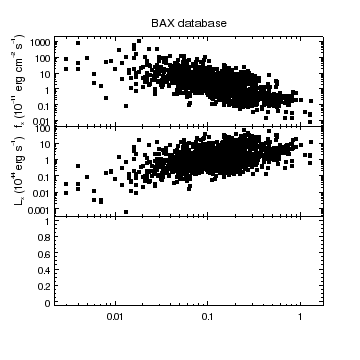### Figure 3: Filling in the middle plot

Since the currency was changed to the middle plot ("plot2") the redshift-luminosity data is drawn there instead of in the top plot.

We finish off adding data to the plot by moving to the last plot (plot3) and plotting up the redshift-temperature data. The output of the info command shows the full set of objects created by the calls; the current objects are the contents of the last plot. The resulting figure is shown in Figure 4.

```chips> current_plot("plot3")
chips> add_curve("bax.fits[cols z,tx]")
chips> log_scale(Y_AXIS)
chips> set_plot_ylabel("T_x (keV)")
chips> set_plot_xlabel("redshift")
chips> print(info())
Window [win1]
Frame [frm1]
Plot [plot1]   (0.15,0.65)  .. (0.90,0.90)
Border bottom [bx1]  top [bx2]  left [by1]  right [by2]
X Axis [ax1]
Y Axis [ay1]
Curve [crv1]
Plot [plot2]   (0.15,0.40)  .. (0.90,0.65)
Border bottom [bx1]  top [bx2]  left [by1]  right [by2]
X Axis [ax1]
Y Axis [ay1]
Curve [crv1]
Plot [plot3]   (0.15,0.15)  .. (0.90,0.40)
Border bottom [bx1]  top [bx2]  left [by1]  right [by2]
X Axis [ax1]
Y Axis [ay1]
Curve [crv1]
```

### Figure 4: Filling in the last plot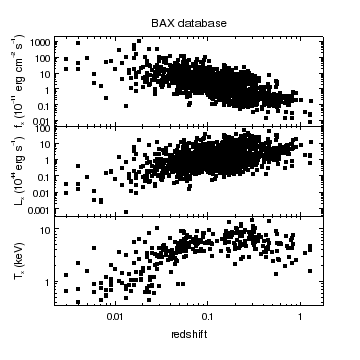### Figure 4: Filling in the last plot

There is now data added to all three plots: flux versus redshift in plot1, luminosity versus redshift in plot2, and temperature versus redshift in plot3.

The grid of plots created by strip_chart can be adjusted to change the spacing between plots (the "gap") and the relative sizes of the plots, as shown below and in Figure 5.

```chips> adjust_grid_ygap(0.05)
chips> adjust_grid_yrelsize(2, 2)
chips> limits(X_AXIS, 0.01, 1.5)
```

### Figure 5: Adjusting the plot sizes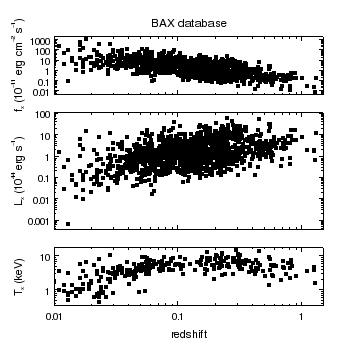### Figure 5: Adjusting the plot sizes

The vertical spacing between the plots, and their relative heights, has been changed by the adjust_grid_ygap and adjust_grid_yrelsize calls.

The single call to limits has changed the X-axis range in all three plots, since the axes are bound together. When an absolute range is used for limits - rather than the "AUTO" value - the axis padding is not applied. So here the X-axis range is 0.01 to 1.5

## Using the optional arguments of strip_chart

The strip_chart has a number of optional argument that allow us to create results like Figure 5. In the code below we set the orientation to 0 (for vertical), gaps value to 0.05, and give an array of relative sizes for the three plots (in this case making the middle plot twice the height of the other plots). The erase command is used to remove the existing frame (frm1 which contained Figure 5) but leave the window. Unlike the clear command - which would have also removed the window - erase does not re-set the number of frames, which is why the frame that is created by strip_chart is called frm2 rather than frm1.

```chips> erase()
chips> print(info())
Window [win1]

chips> strip_chart(3, 0, 0.05, [1,2,1])
chips> print(info())
Window [win1]
Frame [frm2]
Plot [plot1]   (0.15,0.74)  .. (0.90,0.90)
Border bottom [bx1]  top [bx2]  left [by1]  right [by2]
X Axis [ax1]
Y Axis [ay1]
Plot [plot2]   (0.15,0.36)  .. (0.90,0.69)
Border bottom [bx1]  top [bx2]  left [by1]  right [by2]
X Axis [ax1]
Y Axis [ay1]
Plot [plot3]   (0.15,0.15)  .. (0.90,0.31)
Border bottom [bx1]  top [bx2]  left [by1]  right [by2]
X Axis [ax1]
Y Axis [ay1]

chips> add_curve("bax.fits[cols z,fx]")
chips> log_scale()
chips> current_plot("plot2")
chips> add_curve("bax.fits[cols z,lx]")
chips> log_scale()
chips> current_plot("plot3")
chips> add_curve("bax.fits[cols z,tx]")
chips> log_scale()
```

### Figure 6: The optional arguments to strip_chart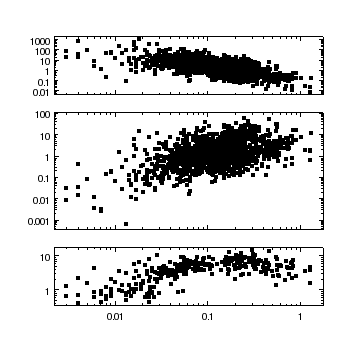### Figure 6: The optional arguments to strip_chart

The plot layout is the same as Figure 5 but this time it was set by the strip_chart call when the plots were created.

## Using the split command to manually create a strip chart

The strip_chart command is a high-level routine that uses a number of ChIPS calls to create a set of strip charts. In this section we show how you can use ChIPS to manually create a plot like Figure 5.

The add_curve command below automatically creates a single plot since there is no current plot at the time of the call. To help identify the plots and data sets we set the id of the curve to fx in the add_curve call. We change the scaling of both axes to logarithmic, and then use the split command to create a second plot, arranged vertically, as shown in Figure 7. Since the split command created a plot - in this case plot2 - it was made current, as shown in the output of info.

```chips> erase()
chips> print(info())
Window [win1]

chips> add_curve("bax.fits[cols z,fx]", ["id", "fx"])
chips> log_scale()
chips> split(2)
chips> print(info())
Window [win1]
Frame [frm3]
Plot [plot1]   (0.15,0.52)  .. (0.90,0.90)
Border bottom [bx1]  top [bx2]  left [by1]  right [by2]
Curve [fx]
X Axis [ax1]
Y Axis [ay1]
Plot [plot2]   (0.15,0.15)  .. (0.90,0.52)
Border bottom [bx1]  top [bx2]  left [by1]  right [by2]

```

### Figure 7: Creating two plots using split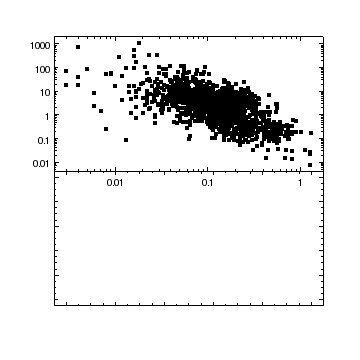### Figure 7: Creating two plots using split

The split command has created a second plot (in the lower half of the frame) and re-sized the existing plot so that it occupies the top-half of the frame.

Note that the second plot does not contain any axes - i.e. no numeric values along the axes - just a border with tick marks. The values of 0.01, 0.1, and 1 come from the X axis of the top plot.

As the second plot is current, any add_curve call will add data to it (and also create a pair of axes). We again create a custom id for the curve, in this case lx; these id values can be seen in the output of the info command below. The resulting visualization is shown in Figure 8.

```chips> add_curve("bax.fits[cols z,lx]", ["id", "lx"])
chips> log_scale()
chips> print(info())
Window [win1]
Frame [frm3]
Plot [plot1]   (0.15,0.52)  .. (0.90,0.90)
Border bottom [bx1]  top [bx2]  left [by1]  right [by2]
Curve [fx]
X Axis [ax1]
Y Axis [ay1]
Plot [plot2]   (0.15,0.15)  .. (0.90,0.52)
Border bottom [bx1]  top [bx2]  left [by1]  right [by2]
Curve [lx]
X Axis [ax1]
Y Axis [ay1]

```

### Figure 8: Adding (z,lx) data to the second plot### Figure 8: Adding (z,lx) data to the second plot

The redshift-luminosity data has been added to the second plot since it was the current plot after the call to split. The add_curve command also created the X and Y axes for this plot. Since we are using the same X-axis data in the two plots, and use the same axis scaling, the tick labels now match up. The tick labels from the top plot can be turned off, so they do not overlap the data in the second plot, by setting the ticklabel.visible attribute of the X axis to 0; an example of this is shown below when creating Figure 11.

In order to add the redshift-temperature data we need another plot, so we use the split command to create a third plot, and automatically move and re-size the existing plots. The result is shown in Figure 9.

```chips> split(3)
chips> print(info())
Window [win1]
Frame [frm3]
Plot [plot1]   (0.15,0.65)  .. (0.90,0.90)
Border bottom [bx1]  top [bx2]  left [by1]  right [by2]
Curve [fx]
X Axis [ax1]
Y Axis [ay1]
Plot [plot2]   (0.15,0.40)  .. (0.90,0.65)
Border bottom [bx1]  top [bx2]  left [by1]  right [by2]
Curve [lx]
X Axis [ax1]
Y Axis [ay1]
Plot [plot3]   (0.15,0.15)  .. (0.90,0.40)
Border bottom [bx1]  top [bx2]  left [by1]  right [by2]

```

### Figure 9: Adding a third plot area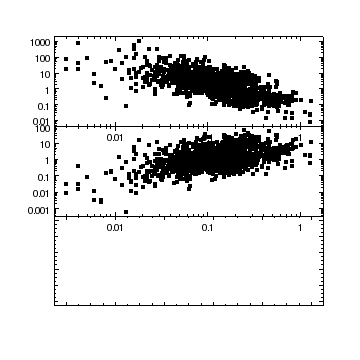### Figure 9: Adding a third plot area

As with Figure 7, the split command has created a new plot and re-sized the existing plots.

The temperature-redshift data is added to the bottom plot, since it is current. The vertical gap between the plots is changed from 0 to 0.05 and the relative heights of the plots changed: in this case adjust_grid_yrelsize(2,2) and adjust_grid_yrelsizes([1,2,1]) have the same effect. The result is Figure 10.

```chips> add_curve("bax.fits[cols z,tx]", ["id", "tx"])
chips> log_scale()
chips> adjust_grid_ygap(0.05)
chips> adjust_grid_yrelsizes([1, 2, 1])
```

### Figure 10: Filling in the third plot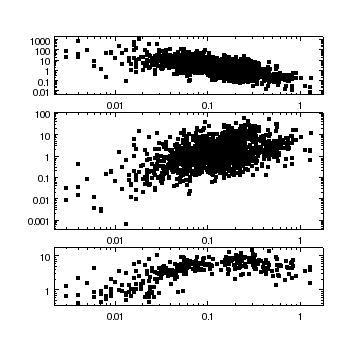### Figure 10: Filling in the third plot

The plot now resembles Figure 6, except that the top two plots still contain the X-axis ticklabels.

To finish the figure, we want to hide the X-axis ticklabels from the first two plots:

```chips> current_plot("plot1")
chips> set_xaxis(["ticklabel.visible", False])
chips> current_plot("plot2")
chips> set_xaxis(["ticklabel.visible", False])
```

We now bind together the X axes of the three plots so that a change to the limits or scaling of one of them will automatically be reflected in all the plots. If the two bind_axes calls had not been made then the limits call would only have changed the last plot.

```chips> bind_axes("plot3", "ax1", "plot1", "ax1")
chips> bind_axes("plot3", "ax1", "plot2", "ax1")
chips> limits(X_AXIS, 0.001, 10)
```

An alternative to the bind_axes call would have been to change the X-axis limits for each plot individually, such as when changing the ticklabel.visible attributes above, or the Y-axis labels below.

We finish by adding labels to the Y axes of the plots, to create Figure 11.

```chips> set_plot_ylabel("plot1", "flux")
chips> set_plot_title("BAX database")
chips> set_plot_ylabel("plot2", "Lx")
chips> set_plot_ylabel("plot3", "Tx")
chips> set_plot_xlabel("redshift")
```

### Figure 11: The final version### Figure 11: The final version

The results are now similar to Figure 5 (the X-axis range and labels on the Y axes are different).

## Creating grids of plots

In this section we shall show how the split command can be used to re-arrange existing plots, rather than create new plots as we did in the previous section.

Starting from Figure 11, we use split to show the first two plots, with the third one hidden from view (as shown in the output of info). Although the last plot (plot3) and its contents were current at the time that Figure 11 was created, the split call has changed the currency to plot2, since plot3 is no longer visible. The results are shown in Figure 12.

```chips> split(2)
chips> print(info())
Window [win1]
Frame [frm3]
Plot [plot1]   (0.15,0.52)  .. (0.90,0.90)
Border bottom [bx1]  top [bx2]  left [by1]  right [by2]
Curve [fx]
X Axis [ax1]
Y Axis [ay1]
Plot [plot2]   (0.15,0.15)  .. (0.90,0.52)
Border bottom [bx1]  top [bx2]  left [by1]  right [by2]
Curve [lx]
X Axis [ax1]
Y Axis [ay1]
Plot [plot3]   (OFFSCREEN)

```

### Figure 12: Hiding the last plot### Figure 12: Hiding the last plot

The first two plots are arranged vertically, and have equal heights and there is no gap between them (so similar to Figure 8). There is no X-axis label of redshift because this label belongs to the last plot (plot3) which has been hidden by the split call.

The behavior of split is to use existing plots if there are any, creating new ones if needed. If different options are needed - such as only use existing plots and do not create new ones - then you will have to use the grid_objects command and set the optional usage argument.

The third plot can be re-displayed by using split again (Figure 13). The currency has been changed back to plot3, now that it is visible once more.

```chips> split(3)
chips> print(info())
Window [win1]
Frame [frm3]
Plot [plot1]   (0.15,0.65)  .. (0.90,0.90)
Border bottom [bx1]  top [bx2]  left [by1]  right [by2]
Curve [fx]
X Axis [ax1]
Y Axis [ay1]
Plot [plot2]   (0.15,0.40)  .. (0.90,0.65)
Border bottom [bx1]  top [bx2]  left [by1]  right [by2]
Curve [lx]
X Axis [ax1]
Y Axis [ay1]
Plot [plot3]   (0.15,0.15)  .. (0.90,0.40)
Border bottom [bx1]  top [bx2]  left [by1]  right [by2]
Curve [tx]
X Axis [ax1]
Y Axis [ay1]

```

### Figure 13: Re-displaying the last plot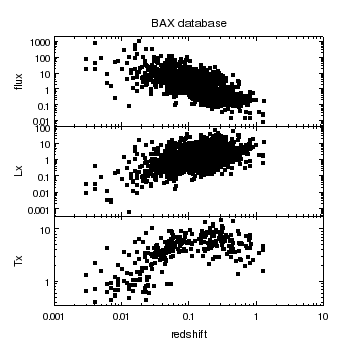### Figure 13: Re-displaying the last plot

All three plots are re-displayed. The output does not match that of Figure 11 since we did not set the optional ygap parameter of split, and split forces the plots to have equal heights.

We now decide to arrange the plots into a two-by-two grid, with no spacing vertically but a small gap horizontally. Note that the plot order goes left to right, top to bottom, so top left is plot1, top right is plot2, bottom left is plot3, and a new plot is created for the bottom right position of the grid (plot4). The resulting visualization is shown in Figure 14.

```chips> split(2, 2, 0, 0.05)
chips> print(info())
Window [win1]
Frame [frm3]
Plot [plot1]   (0.15,0.52)  .. (0.50,0.90)
Border bottom [bx1]  top [bx2]  left [by1]  right [by2]
Curve [fx]
X Axis [ax1]
Y Axis [ay1]
Plot [plot2]   (0.55,0.52)  .. (0.90,0.90)
Border bottom [bx1]  top [bx2]  left [by1]  right [by2]
Curve [lx]
X Axis [ax1]
Y Axis [ay1]
Plot [plot3]   (0.15,0.15)  .. (0.50,0.52)
Border bottom [bx1]  top [bx2]  left [by1]  right [by2]
Curve [tx]
X Axis [ax1]
Y Axis [ay1]
Plot [plot4]   (0.55,0.15)  .. (0.90,0.52)
Border bottom [bx1]  top [bx2]  left [by1]  right [by2]

```

### Figure 14: A two-by-two grid of plots### Figure 14: A two-by-two grid of plots

The plots have been arranged left-to-right, top-to-bottom, in a two-by-two grid.

Since the first plot (plot1) has the plot title, it appears over this plot rather than the whole grid. We will change this later when creating Figure 16.

We now remove the fourth plot and swap the positions of the luminosity-redshift (plot2) and temperature-redshift (plot3) graphs to create Figure 15.

```chips> delete_plot()
chips> id = ChipsId()
chips> id.plot = "plot2"
chips> swap_object_positions(id, "plot3")
chips> print(info())
Window [win1]
Frame [frm3]
Plot [plot1]   (0.15,0.52)  .. (0.50,0.90)
Border bottom [bx1]  top [bx2]  left [by1]  right [by2]
Curve [fx]
X Axis [ax1]
Y Axis [ay1]
Plot [plot2]   (0.15,0.15)  .. (0.50,0.52)
Border bottom [bx1]  top [bx2]  left [by1]  right [by2]
Curve [lx]
X Axis [ax1]
Y Axis [ay1]
Plot [plot3]   (0.55,0.52)  .. (0.90,0.90)
Border bottom [bx1]  top [bx2]  left [by1]  right [by2]
Curve [tx]
X Axis [ax1]
Y Axis [ay1]

```

### Figure 15: Swapping plot positions in a grid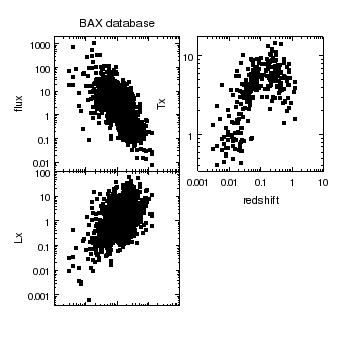### Figure 15: Swapping plot positions in a grid

The locations of the temperture-redshift and luminosity-redshift plots have been swapped, as can be seen by comparing this figure to Figure 14 or the plot coordinates from the output of the info command; before the swap we had:

```    Plot [plot2]   (0.55,0.52)  .. (0.90,0.90)
Plot [plot3]   (0.15,0.15)  .. (0.50,0.52)
```

which became, after the swap,

```    Plot [plot2]   (0.15,0.15)  .. (0.50,0.52)
Plot [plot3]   (0.55,0.52)  .. (0.90,0.90)
```

We finish off the figure by adding and adjusting labels. First we move to plot3 (temperature) and hide the ticklabels on the left Y axis, by setting ticklabel.visible to 0. Changing the offset.perpendicular attribute of the Y axis moves the label into the gap between the plots. We then turn on the ticklabels of the right-hand Y axis (this is the border called by2 in the info output).

```chips> current_plot("plot3")
chips> set_yaxis(["ticklabel.visible", False])
chips> set_yaxis(["offset.perpendicular", 5])
chips> set_yaxis("by2", ["ticklabel.visible", True])
```

To give a bit more space between the columns we increase the X gap to 0.07 (it was 0.05) and decide to increase the spacing between the "Tx" label and the axis to take advantage of this extra room.

```chips> adjust_grid_xgap(0.07)
chips> set_yaxis(["offset.perpendicular", 7])
```

We now move to the bottom-left plot (plot2, which plots luminosity versus redshift) and get the X-axis ticklabels to be displayed, and also add the "redshift" label to this axis.

```chips> current_plot("plot2")
chips> set_xaxis(["ticklabel.visible", True])
chips> set_plot_xlabel("redshift")
```

Finally we want to have the title be centered on the grid. To do this we first remove the existing title (which belongs to the plot called plot1), then we add a label using the frame-normalized coordinate system (this is the same system used to report the plot positions in the info output as discussed in the caption to Figure 15). We use an X position of 0.5 and set the horiozontal-alignment attribute halign to 0.5 so that the label is horizontally centered on this position. The Y coordinate is set to 0.93 since we know that the plots end at y=0.9. Finally, we increase the label size, and end up with Figure 16.

```chips> set_plot_title("plot1", "")
chips> add_label(0.5, 0.93, "BAX database", ["id", "title", "coordsys", FRAME_NORM])
chips> set_label(["size", 18, "halign", 0.5])
chips> print(info())
Window [win1]
Frame [frm3]
Plot [plot1]   (0.15,0.52)  .. (0.49,0.90)
Border bottom [bx1]  top [bx2]  left [by1]  right [by2]
Curve [fx]
X Axis [ax1]
Y Axis [ay1]
Label [title]
Plot [plot2]   (0.15,0.15)  .. (0.49,0.52)
Border bottom [bx1]  top [bx2]  left [by1]  right [by2]
Curve [lx]
X Axis [ax1]
Y Axis [ay1]
Plot [plot3]   (0.56,0.52)  .. (0.90,0.90)
Border bottom [bx1]  top [bx2]  left [by1]  right [by2]
Curve [tx]
X Axis [ax1]
Y Axis [ay1]

```

### Figure 16: The final grid of plots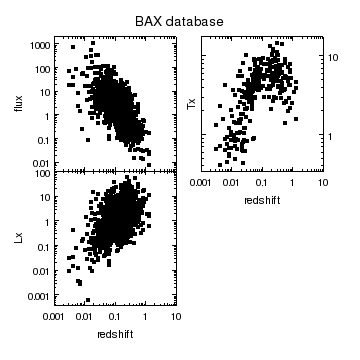### Figure 16: The final grid of plots

The final finishing touches have been applied to Figure 15. The axis labels, both text and numeric, have been adjusted and a title label added to the grid.

## Summary

• The low-level command for creating grids of plots is grid_objects but for most cases the higher-level routines split and strip_chart are to be preferred. The strip_chart command is useful when you want to create a single column or row of plots which share a common axis, whereas the split command can create a n by m grid of plots (n and m can be 1).

• Once a grid of plots has been created - using any of the three commands above - the spacing, relative sizes (width and height), and positions within the grid can be changed.

• Axes can be bound together so that changes in the range (limits) or scaling (log_scale and lin_scale) of any one of the set will be automatically reflected in the others in the set. This is useful when you have multiple plots and you want to keep an axis lined up (which is why strip_chart automatically binds the common axis for all the plots it creates), or if you want to add extra axes to a plot and keep them all aligned.

• The info_current command shows the current set of objects. By setting the info.current preference setting to true you can also get this information included in the output of the info command (as reverse-video text, shown in bold in the examples above).

The output of the info command includes the positions of the bottom-left and top-right corners of each plot, which can be useful when trying to work out what plot is where. The output

```    Plot [plot1]   (0.15,0.52)  .. (0.50,0.90)
Plot [plot2]   (0.55,0.52)  .. (0.90,0.90)
Plot [plot3]   (0.15,0.15)  .. (0.50,0.52)
Plot [plot4]   (0.55,0.15)  .. (0.90,0.52)
```

says that plot1 is in the top-left corner (x goes from 0.15 to 0.5 and y from 0.52 to 0.9) and plot4 is in the bottom-right corner (since x spans 0.55 - 0.9 and y spans 0.15 - 0.52).

• Labels and other annotations - such as lines, points, and regions, can be positioned using multiple coordinate systems. By using the coordsys attribute, you can say

```chips> add_label(0.5, 0.5, "A Label", ["coordsys", FRAME_NORM, "halign", 0.5, "valign", 0.5])
```

and the label will be centered - both horizontally and vertically - within the frame. If the PLOT_NORM coordinate system had been used instead, and a plot already existed, then the label would have been centered in the plot and would not move if the axis limits were changed.

• The split and grid_objects commands can temporarily hide excess plots, as shown when creating Figure 12. The hide_plot and display_plot commands can also be used to change the visibility of plots.

## History

 09 Jul 2008 New for CIAO 4.0 15 Dec 2008 CIAO 4.1 - the coordsys attribute can be used to position annotations such as labels 15 Dec 2009 Updated for CIAO 4.2: added PDF versions of all plots. 15 Dec 2010 Updated for CIAO 4.3: the info output has changed slightly; the order of objects within a plot may have changed and the auto-generated names of coordinate systems has cahnged from strings like "plot1_ax1ay2" to ones like "ds2.2.3.13". 15 Dec 2011 Updated for CIAO 4.4. 13 Dec 2012 Updated for CIAO 4.5. 05 Dec 2013 Updated for CIAO 4.6. 10 Dec 2014 Updated for CIAO 4.7. 15 Dec 2015 Updated for CIAO 4.8.